# NCERT Solutions for Class 10 Science Chapter 1

NCERT Solutions for Class 10 Science Chapter 1 – Chemical Reactions and Equations, contains solutions to various questions in Exercises for Chapter 1. Chemical Reactions and Equations Class 10 NCERT Solutions have been explained in a simple and easy to understand manner. We are providing all subjects which can be accessed by clicking here.

## Download NCERT Solutions for Class 10 Science Chapter 1 – Chemical Reactions and Equations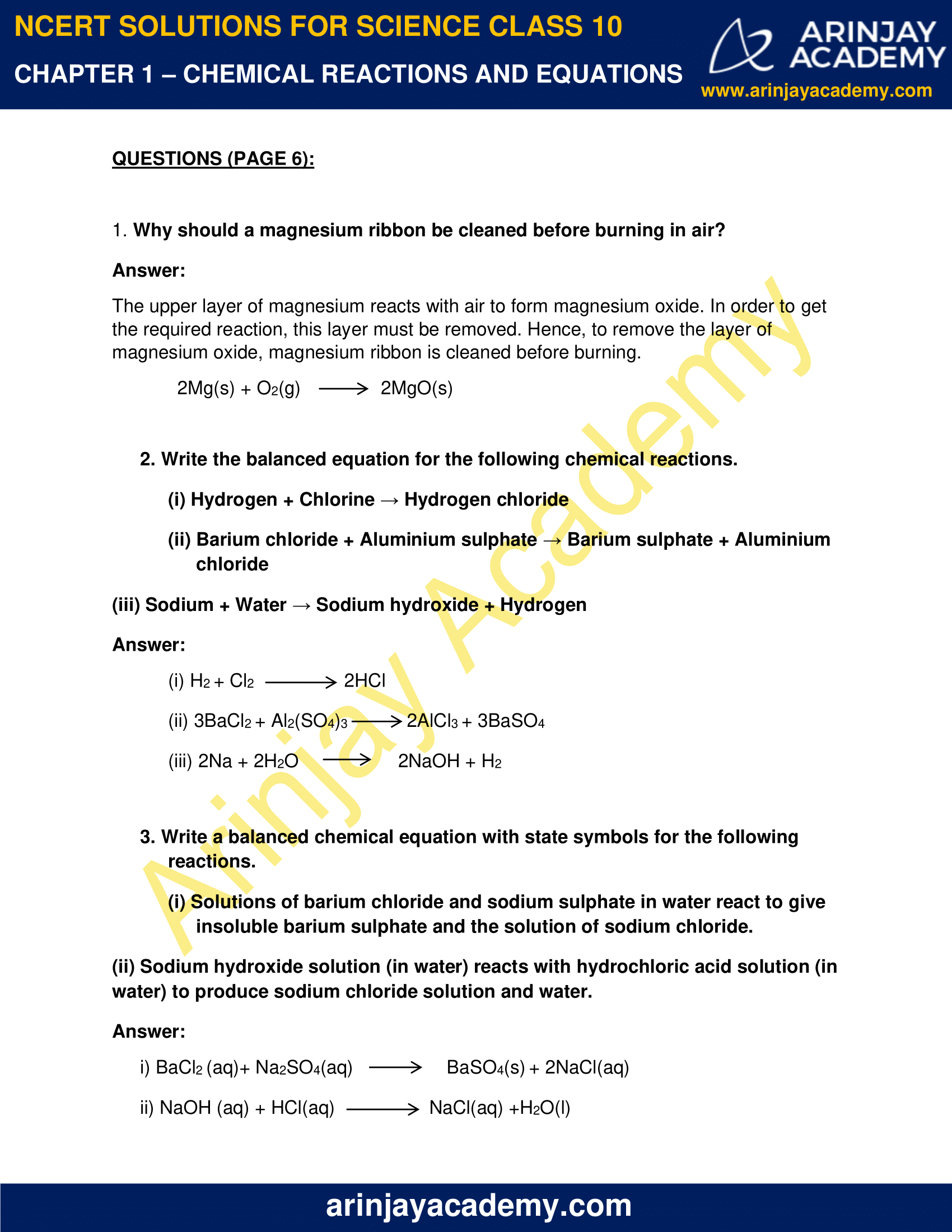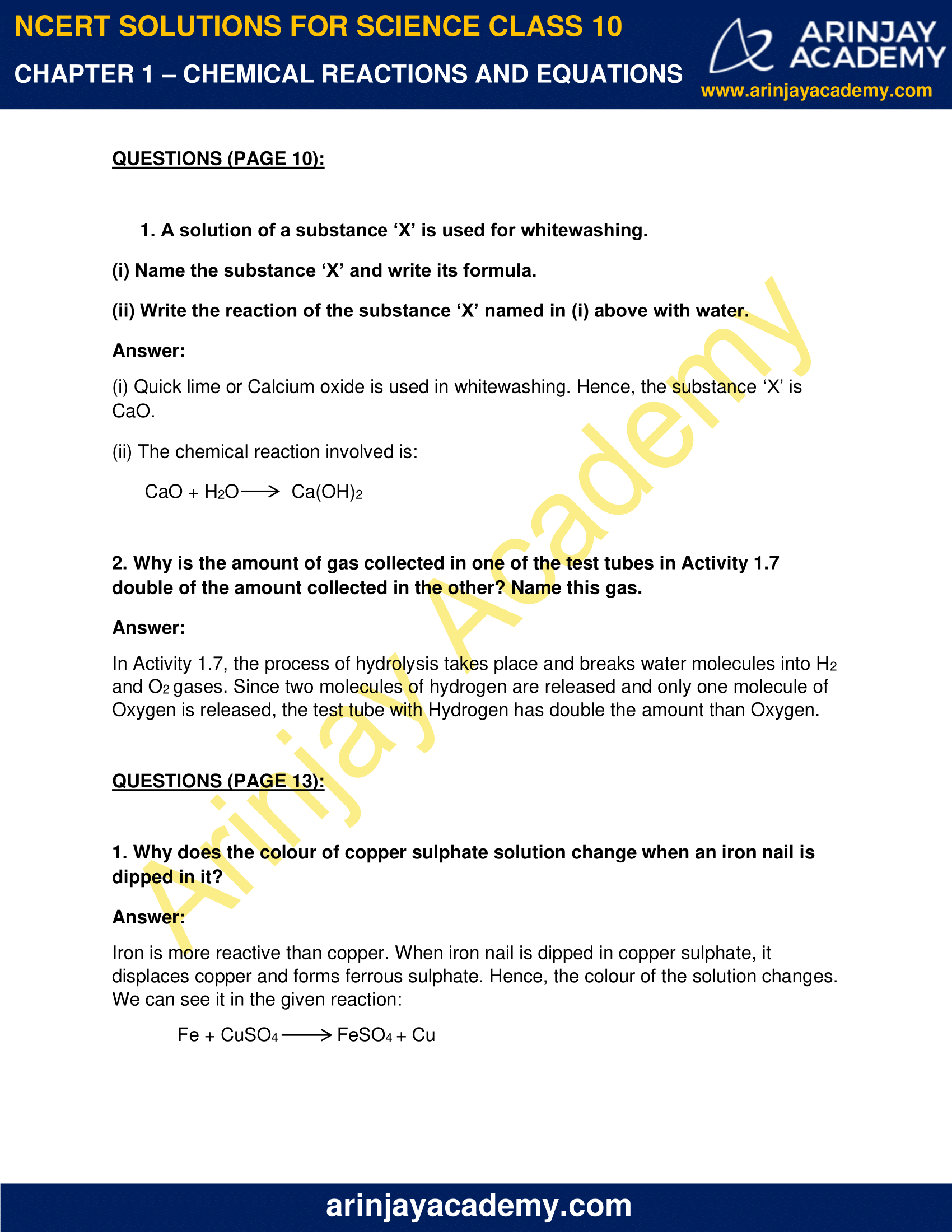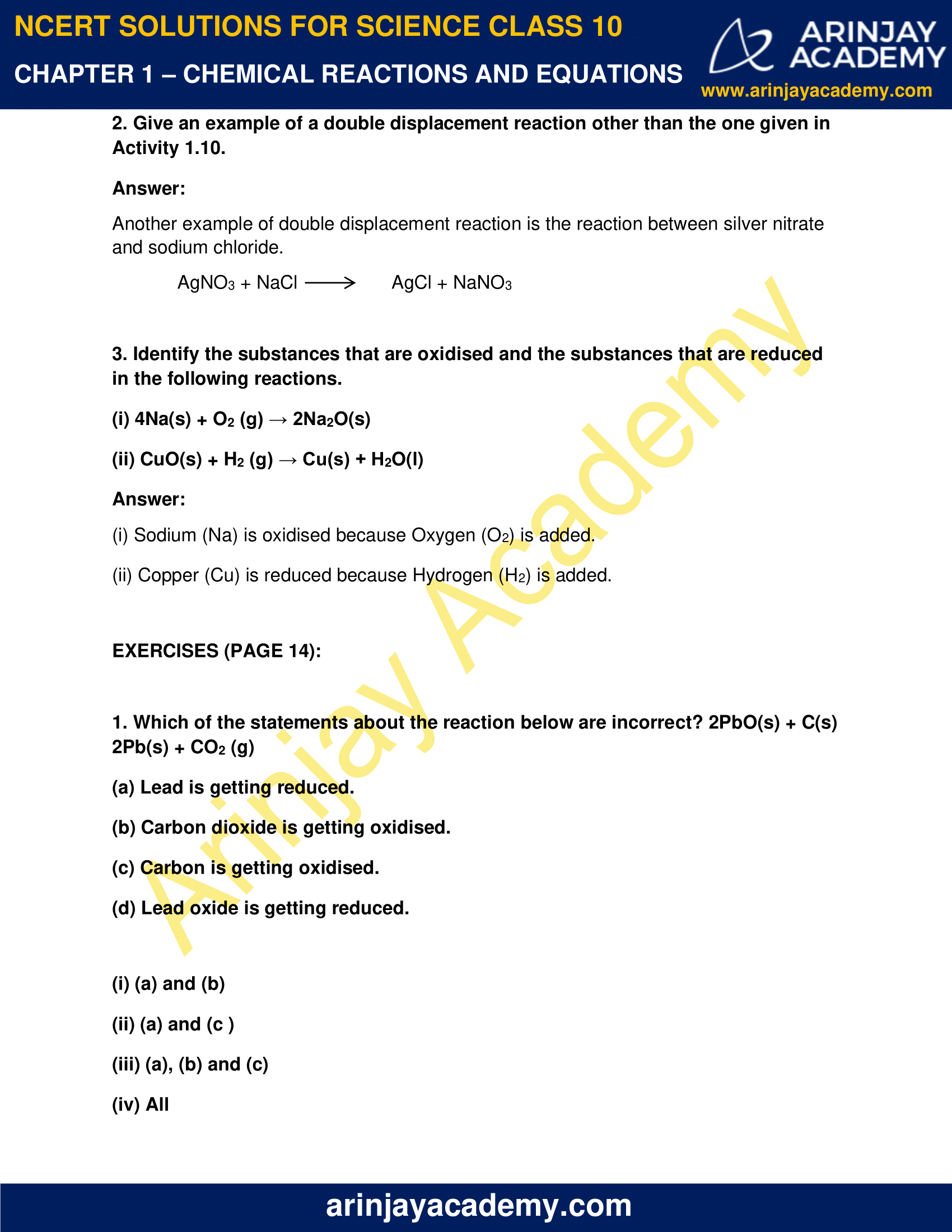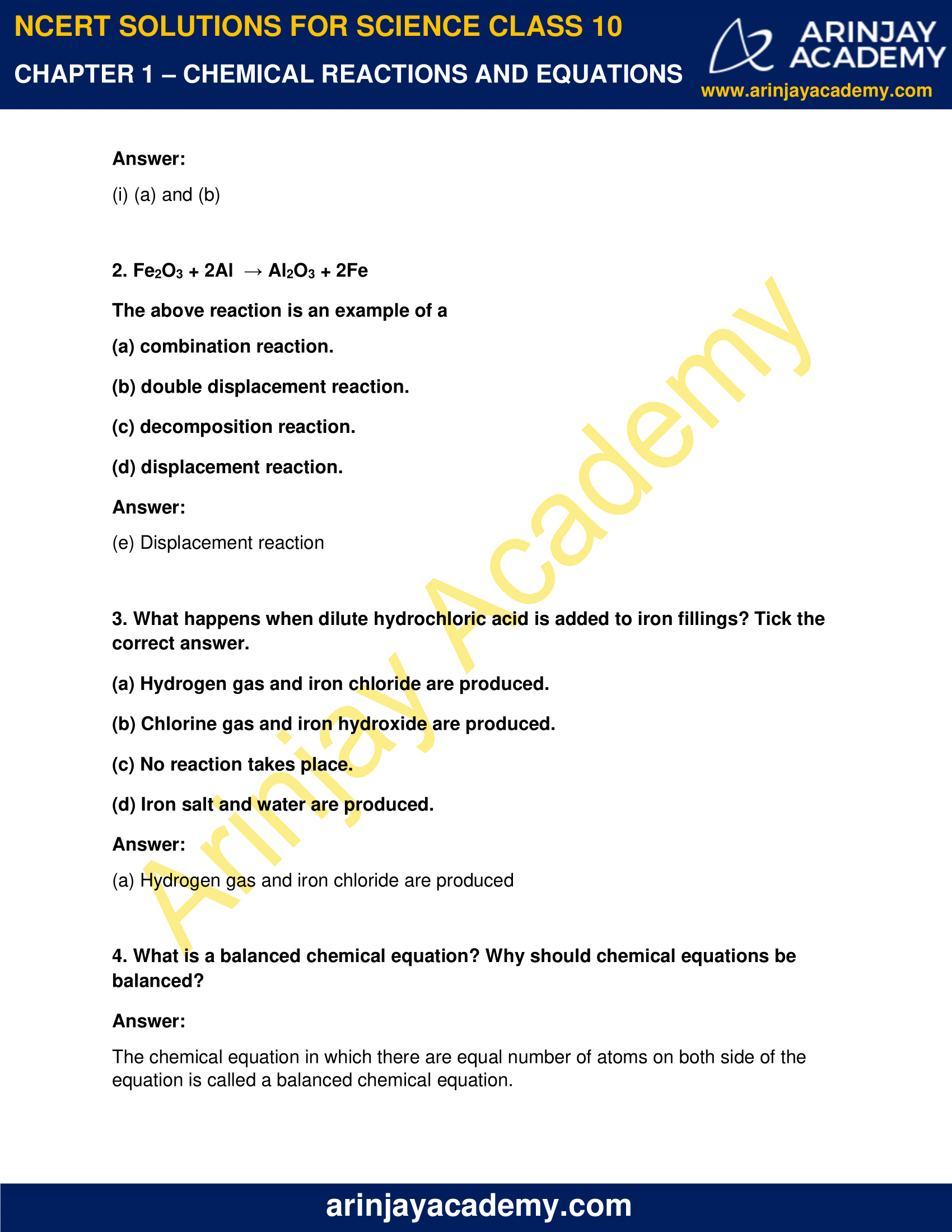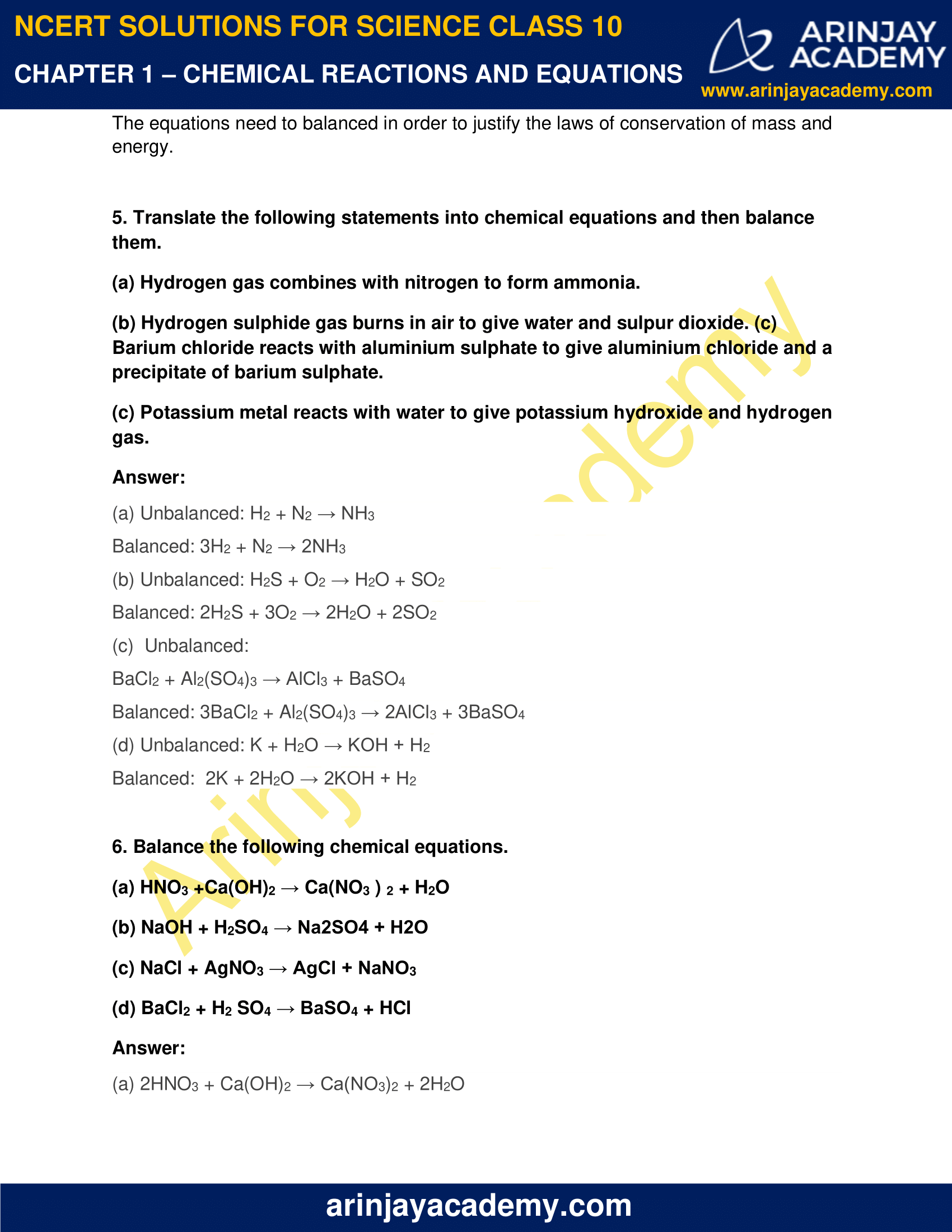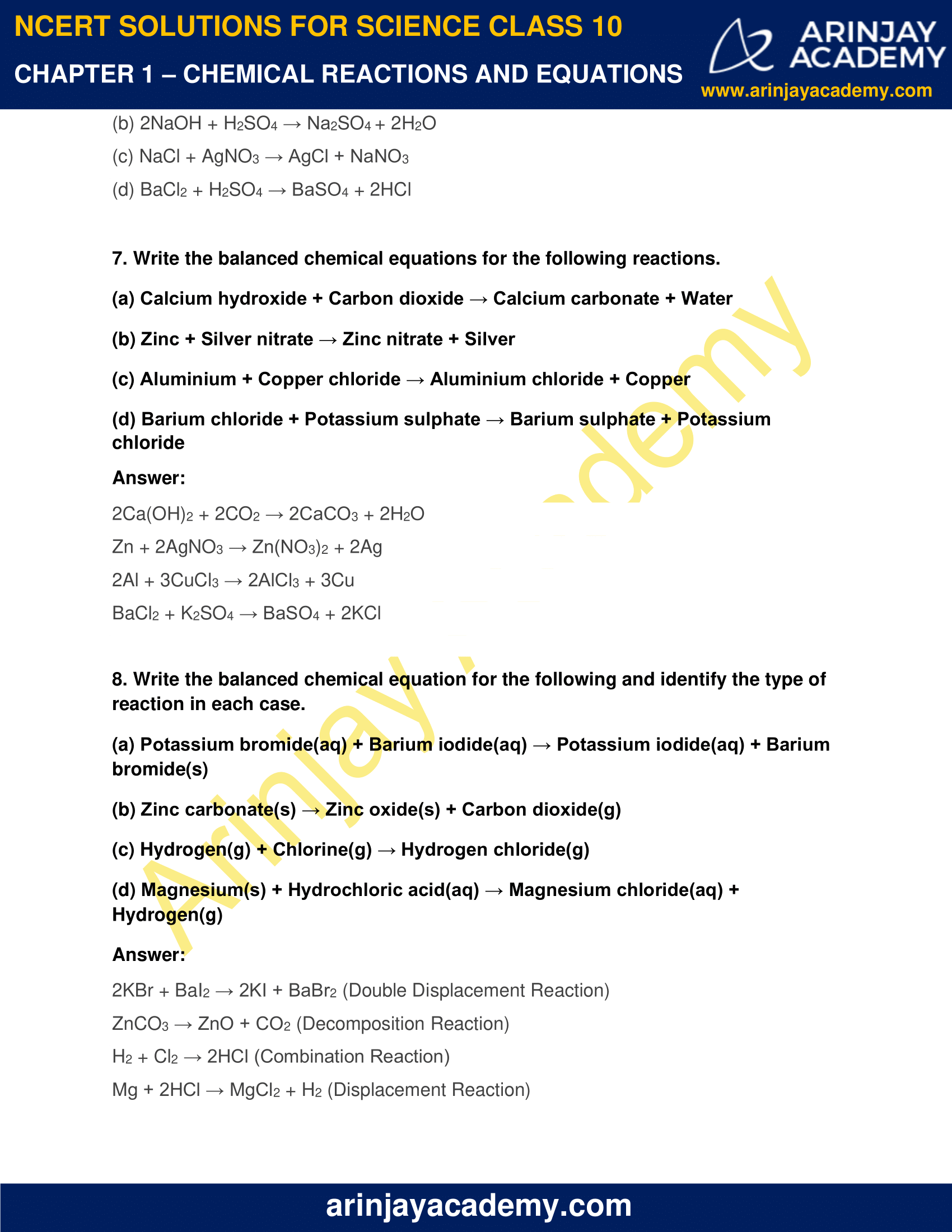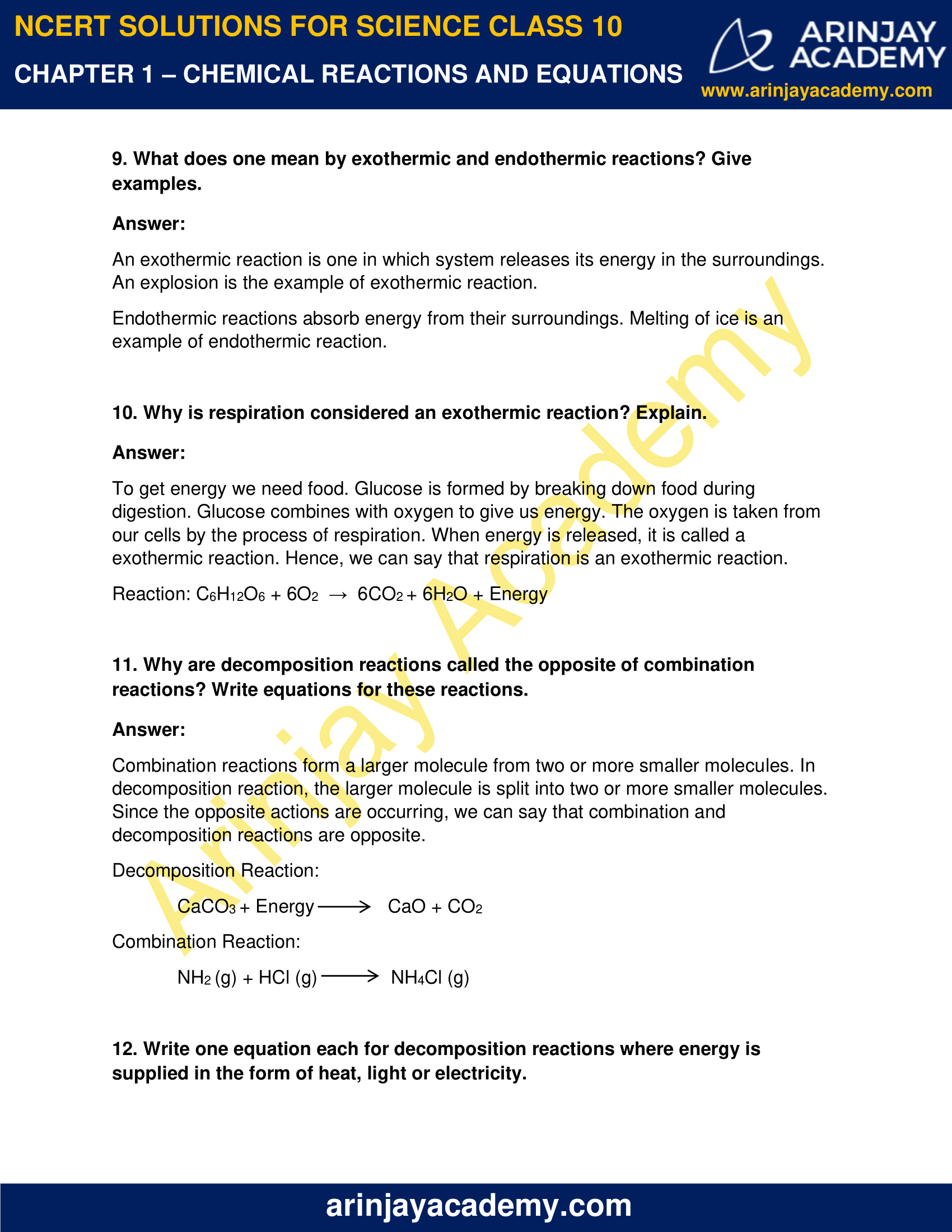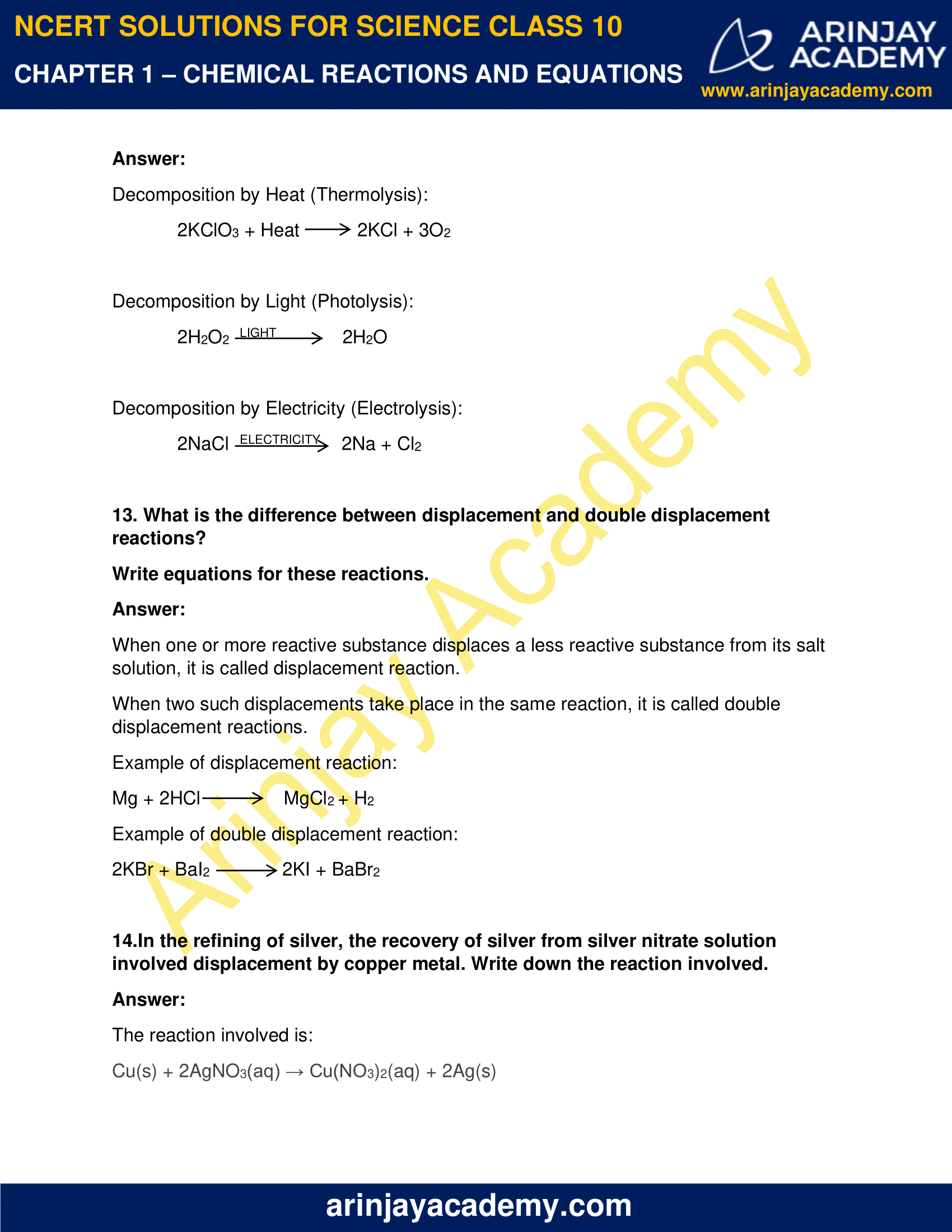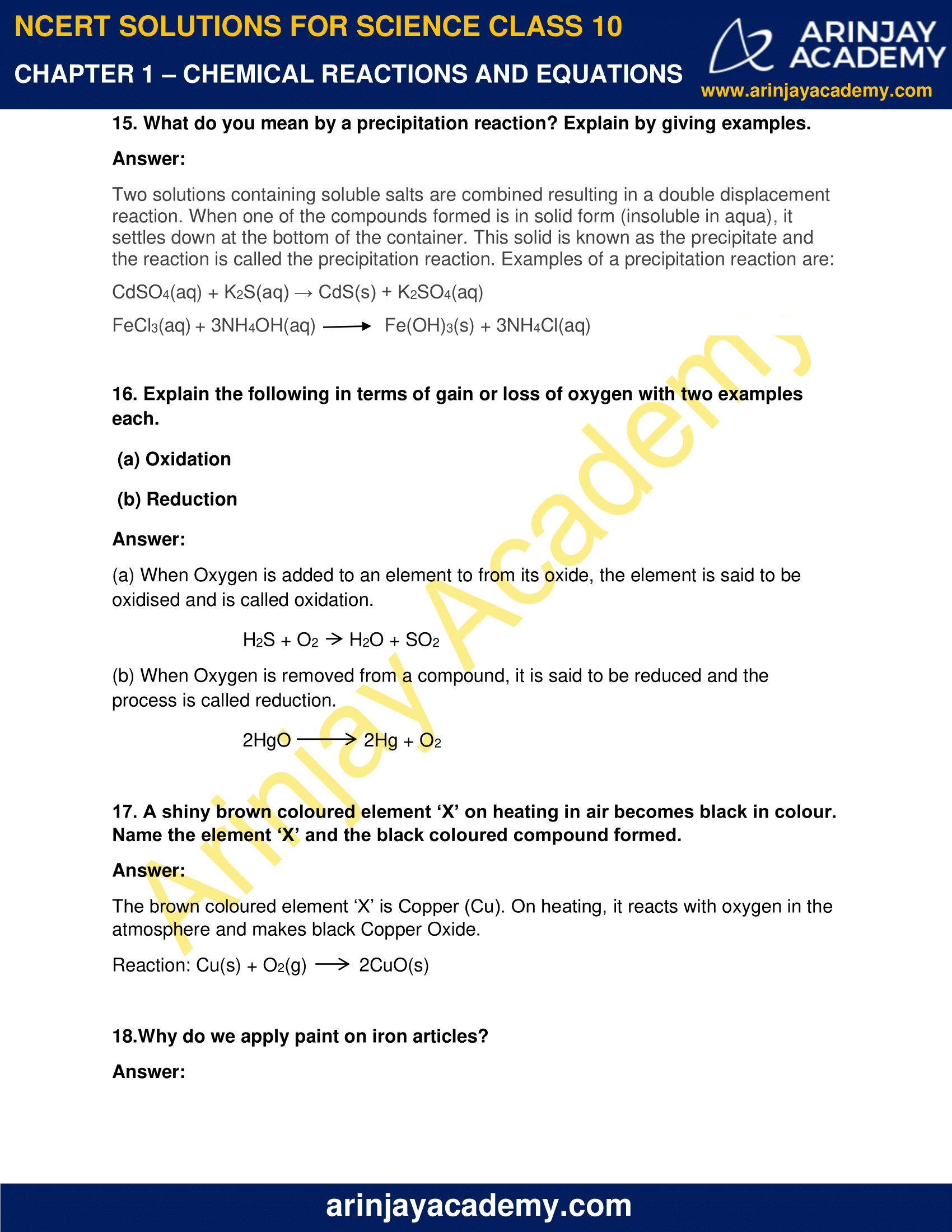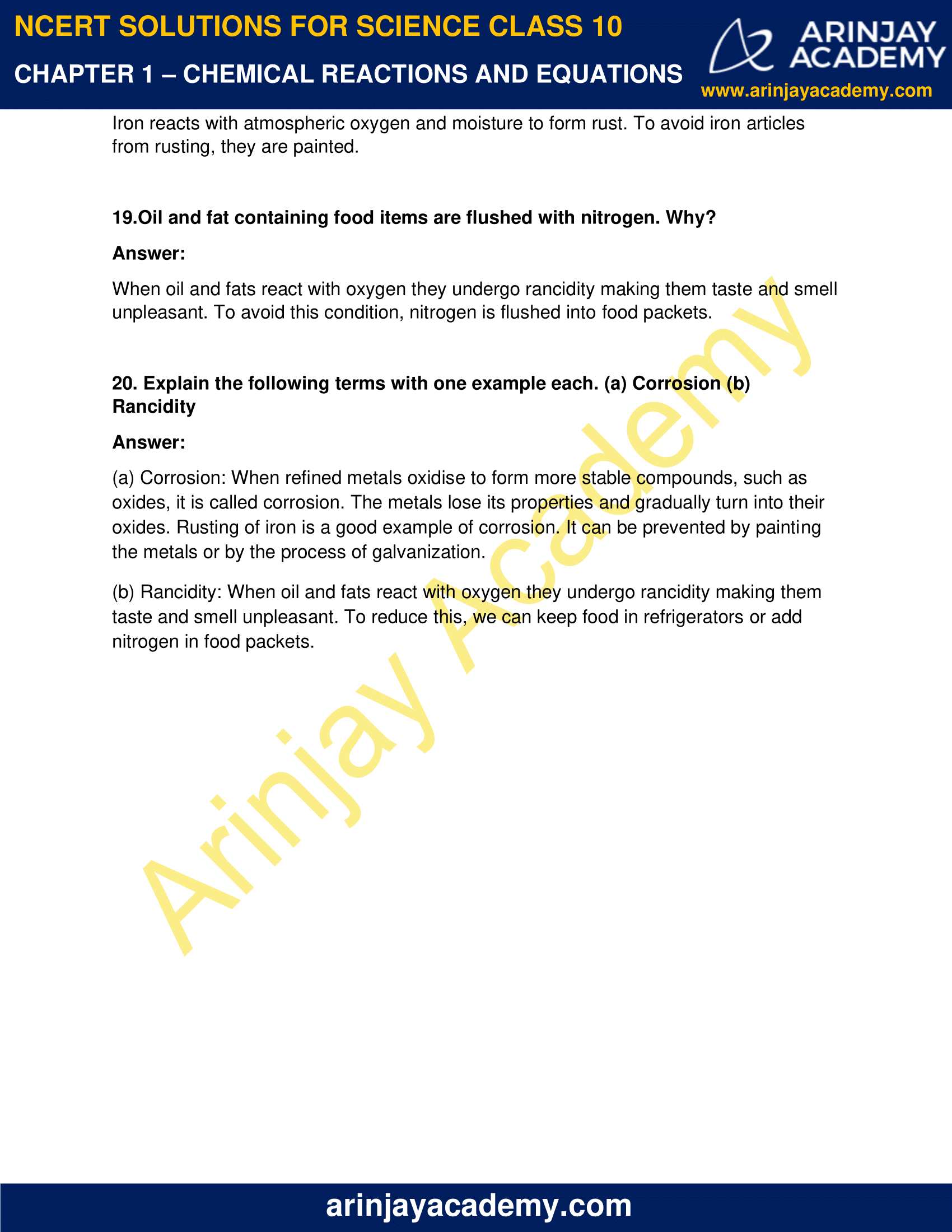### NCERT Solutions for Class 10 Science Chapter 1 – Chemical Reactions and Equations – NCERT Exercises

NCERT Solutions for Class 10 Science Chapter 1 – Chemical Reactions and Equations, Exercises includes all in text and exercise solved questions which helps you to understand the topic covered in Chapter 1 Chemical Reactions and Equations Class 10, in a better manner to help you to score good marks in your examinations.

### NCERT Solutions for Class 10 Science Chapter 1 – Intext Questions – Page 6

1. Why should a magnesium ribbon be cleaned before burning in air?

The upper layer of magnesium reacts with air to form magnesium oxide. In order to get the required reaction, this layer must be removed. Hence, to remove the layer of magnesium oxide, magnesium ribbon is cleaned before burning.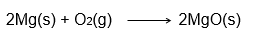2. Write the balanced equation for the following chemical reactions.

(i) Hydrogen + Chlorine → Hydrogen chloride

(ii) Barium chloride + Aluminium sulphate → Barium sulphate + Aluminium chloride

(iii) Sodium + Water → Sodium hydroxide + Hydrogen

(i) H2 + Cl2 2HCl

(ii) 3BaCl2 + Al2(SO4)3 2AlCl3 + 3BaSO4

(iii) 2Na + 2H2O  2NaOH + H2

3. Write a balanced chemical equation with state symbols for the following reactions.

(i) Solutions of barium chloride and sodium sulphate in water react to give insoluble barium sulphate and the solution of sodium chloride.

(ii) Sodium hydroxide solution (in water) reacts with hydrochloric acid solution (in water) to produce sodium chloride solution and water.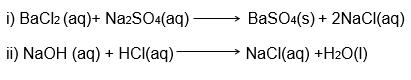### Intext Questions – Page 10

1. A solution of a substance ‘X’ is used for whitewashing.

(i) Name the substance ‘X’ and write its formula.

(ii) Write the reaction of the substance ‘X’ named in (i) above with water.

(i) Quick lime or Calcium oxide is used in whitewashing. Hence, the substance ‘X’ is CaO.

(ii) The chemical reaction involved is:

CaO + H2O ⇒ Ca(OH)2

2. Why is the amount of gas collected in one of the test tubes in Activity 1.7 double of the amount collected in the other? Name this gas.

In Activity 1.7, the process of hydrolysis takes place and breaks water molecules into H2 and O2 gases. Since two molecules of hydrogen are released and only one molecule of Oxygen is released, the test tube with Hydrogen has double the amount than Oxygen.

### Intext Questions – Page 13

1. Why does the colour of copper sulphate solution change when an iron nail is dipped in it?

Iron is more reactive than copper. When iron nail is dipped in copper sulphate, it displaces copper and forms ferrous sulphate. Hence, the colour of the solution changes. We can see it in the given reaction:

Fe + CuSO⇒ FeSO4 + Cu

2. Give an example of a double displacement reaction other than the one given in Activity 1.10.

Another example of double displacement reaction is the reaction between silver nitrate and sodium chloride.

AgNO3 + NaCl ⇒ AgCl + NaNO3

3. Identify the substances that are oxidised and the substances that are reduced in the following reactions.

(i) 4Na(s) + O2 (g) → 2Na2O(s)

(ii) CuO(s) + H2 (g) → Cu(s) + H2O(l)

(i) Sodium (Na) is oxidised because Oxygen (O2) is added.

(ii) Copper (Cu) is reduced because Hydrogen (H2) is added.

### NCERT Solutions for Class 10 Science Chapter 1 – NCERT Exercise – Page 14

1. Which of the statements about the reaction below are incorrect?

2PbO(s) + C(s) ⇒ 2Pb(s) + CO2 (g)

(b) Carbon dioxide is getting oxidised.
(c) Carbon is getting oxidised.
(d) Lead oxide is getting reduced.

(i) (a) and (b)
(ii) (a) and (c )
(iii) (a), (b) and (c)
(iv) All

(i) (a) and (b)

2. Fe2O3 + 2Al → Al2O3 + 2Fe

The above reaction is an example of a

(a) combination reaction.
(b) double displacement reaction.
(c) decomposition reaction.
(d) displacement reaction.

(d) Displacement reaction

3. What happens when dilute hydrochloric acid is added to iron fillings? Tick the correct answer.

(a) Hydrogen gas and iron chloride are produced.

(b) Chlorine gas and iron hydroxide are produced.

(c) No reaction takes place.

(d) Iron salt and water are produced.

(a) Hydrogen gas and iron chloride are produced

4. What is a balanced chemical equation? Why should chemical equations be balanced?

The chemical equation in which there are equal number of atoms on both side of the equation is called a balanced chemical equation.

The equations need to balanced in order to justify the laws of conservation of mass and energy.

5. Translate the following statements into chemical equations and then balance them.

(a) Hydrogen gas combines with nitrogen to form ammonia.

(b) Hydrogen sulphide gas burns in air to give water and sulpur dioxide.

(c) Barium chloride reacts with aluminium sulphate to give aluminium chloride and a precipitate of barium sulphate.

(d) Potassium metal reacts with water to give potassium hydroxide and hydrogen gas.

(a) Unbalanced: H2 + N2 → NH3

Balanced: 3H2 + N2 → 2NH3

(b) Unbalanced: H2S + O2 → H2O + SO2

Balanced: 2H2S + 3O2 → 2H2O + 2SO2

(c)  Unbalanced:

BaCl2 + Al2(SO4)3 → AlCl3 + BaSO4

Balanced: 3BaCl2 + Al2(SO4)3 → 2AlCl3 + 3BaSO4

(d) Unbalanced: K + H2O → KOH + H2

Balanced:  2K + 2H2O → 2KOH + H2

6. Balance the following chemical equations.

(a) HNO3 +Ca(OH)2 → Ca(NO3 ) 2 + H2O
(b) NaOH + H2SO4 → Na2SO4 + H2O
(c) NaCl + AgNO3 → AgCl + NaNO3
(d) BaCl2 + H2 SO4 → BaSO4 + HCl

(a) 2HNO3 + Ca(OH)2 → Ca(NO3)2 + 2H2O
(b) 2NaOH + H2SO4 → Na2SO+ 2H2O
(c) NaCl + AgNO3 → AgCl + NaNO3
(d) BaCl2 + H2SO4 → BaSO4 + 2HCl

7. Write the balanced chemical equations for the following reactions.

(a) Calcium hydroxide + Carbon dioxide → Calcium carbonate + Water
(b) Zinc + Silver nitrate → Zinc nitrate + Silver
(c) Aluminium + Copper chloride → Aluminium chloride + Copper
(d) Barium chloride + Potassium sulphate → Barium sulphate + Potassium chloride

(a) 2Ca(OH)2 + 2CO2 → 2CaCO3 + 2H2O
(b) Zn + 2AgNO3 → Zn(NO3)2 + 2Ag
(c) 2Al + 3CuCl3 → 2AlCl3 + 3Cu
(d) BaCl2 + K2SO4 → BaSO4 + 2KCl

8. Write the balanced chemical equation for the following and identify the type of reaction in each case.

(a) Potassium bromide(aq) + Barium iodide(aq) → Potassium iodide(aq) + Barium bromide(s)
(b) Zinc carbonate(s) → Zinc oxide(s) + Carbon dioxide(g)
(c) Hydrogen(g) + Chlorine(g) → Hydrogen chloride(g)
(d) Magnesium(s) + Hydrochloric acid(aq) → Magnesium chloride(aq) + Hydrogen(g)

(a) 2KBr + BaI2 → 2KI + BaBr2 (Double Displacement Reaction)
(b) ZnCO3 → ZnO + CO2 (Decomposition Reaction)
(c) H2 + Cl2 → 2HCl (Combination Reaction)
(d) Mg + 2HCl → MgCl2 + H2 (Displacement Reaction)

9. What does one mean by exothermic and endothermic reactions? Give examples.

An exothermic reaction is one in which system releases its energy in the surroundings. An explosion is the example of exothermic reaction.

Endothermic reactions absorb energy from their surroundings. Melting of ice is an example of endothermic reaction.

10. Why is respiration considered an exothermic reaction? Explain.

To get energy we need food. Glucose is formed by breaking down food during digestion. Glucose combines with oxygen to give us energy. The oxygen is taken from our cells by the process of respiration. When energy is released, it is called a exothermic reaction. Hence, we can say that respiration is an exothermic reaction.

Reaction: C6H12O6 + 6O2      6CO2 + 6H2O + Energy

11. Why are decomposition reactions called the opposite of combination reactions? Write equations for these reactions.

Combination reactions form a larger molecule from two or more smaller molecules. In decomposition reaction, the larger molecule is split into two or more smaller molecules. Since the opposite actions are occurring, we can say that combination and decomposition reactions are opposite.

Decomposition Reaction:

CaCO3 + Energy ⇒ CaO + CO2

Combination Reaction:

NH2 (g) + HCl (g) ⇒ NH4Cl (g)

12. Write one equation each for decomposition reactions where energy is supplied in the form of heat, light or electricity.

Decomposition by Heat (Thermolysis):

2KClO3 + Heat  ⇒ 2KCl + 3O2

Decomposition by Light (Photolysis):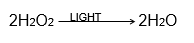Decomposition by Electricity (Electrolysis):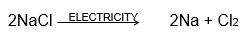13. What is the difference between displacement and double displacement reactions?

Write equations for these reactions.

When one or more reactive substance displaces a less reactive substance from its salt solution, it is called displacement reaction.

When two such displacements take place in the same reaction, it is called double displacement reactions.

Example of displacement reaction:

Mg + 2HCl ⇒ MgCl2 + H2

Example of double displacement reaction:

2KBr + BaI2 ⇒ 2KI + BaBr2

14.In the refining of silver, the recovery of silver from silver nitrate solution involved displacement by copper metal. Write down the reaction involved.

The reaction involved is: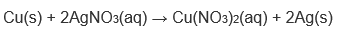15. What do you mean by a precipitation reaction? Explain by giving examples.

Two solutions containing soluble salts are combined resulting in a double displacement reaction. When one of the compounds formed is in solid form (insoluble in aqua), it settles down at the bottom of the container. This solid is known as the precipitate and the reaction is called the precipitation reaction. Examples of a precipitation reaction are: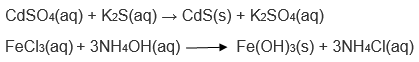16. Explain the following in terms of gain or loss of oxygen with two examples each.

(a) Oxidation
(b) Reduction

(a) When Oxygen is added to an element to from its oxide, the element is said to be oxidised and is called oxidation.

H2S + O2 ⇒ H2O + SO2

(b) When Oxygen is removed from a compound, it is said to be reduced and the process is called reduction.

2HgO ⇒ 2Hg + O2

17. A shiny brown coloured element ‘X’ on heating in air becomes black in colour. Name the element ‘X’ and the black coloured compound formed.

The brown coloured element ‘X’ is Copper (Cu). On heating, it reacts with oxygen in the atmosphere and makes black Copper Oxide.

Reaction:

Cu(s) + O2(g) ⇒ 2CuO(s)

18.Why do we apply paint on iron articles?

Iron reacts with atmospheric oxygen and moisture to form rust. To avoid iron articles from rusting, they are painted.

19.Oil and fat containing food items are flushed with nitrogen. Why?

When oil and fats react with oxygen they undergo rancidity making them taste and smell unpleasant. To avoid this condition, nitrogen is flushed into food packets.

20. Explain the following terms with one example each. (a) Corrosion (b) Rancidity

(a) Corrosion: When refined metals oxidise to form more stable compounds, such as oxides, it is called corrosion. The metals lose its properties and gradually turn into their oxides. Rusting of iron is a good example of corrosion. It can be prevented by painting the metals or by the process of galvanization.

(b) Rancidity: When oil and fats react with oxygen they undergo rancidity making them taste and smell unpleasant. To reduce this, we can keep food in refrigerators or add nitrogen in food packets.

### Topics Covered in Chemical Reactions and Equations Class 10 Science

• Chemical Equations
• Writing a Chemical Equation
• Balanced Chemical Equations
• Types of Chemical Reactions
• Combination Reaction
• Decomposition Reaction
• Displacement Reaction
• Double Displacement Reaction
• Oxidation and Reduction
• Oxidation Reactions